Topics: Coronavirus | AI | Data Science | Deep Learning | Machine Learning | Python | R | Statistics

KDnuggets Home » News » 2016 » Aug » Tutorials, Overviews » The 10 Algorithms Machine Learning Engineers Need to Know ( 16:n31 )

#The 10 Algorithms Machine Learning Engineers Need to Know

http likes 9583

Read this introductory list of contemporary machine learning algorithms of importance that every engineer should understand.

6. Ensemble Methods: Ensemble methods are learning algorithms that construct a set of classifiers and then classify new data points by taking a weighted vote of their predictions. The original ensemble method is Bayesian averaging, but more recent algorithms include error-correcting output coding, bagging, and boosting.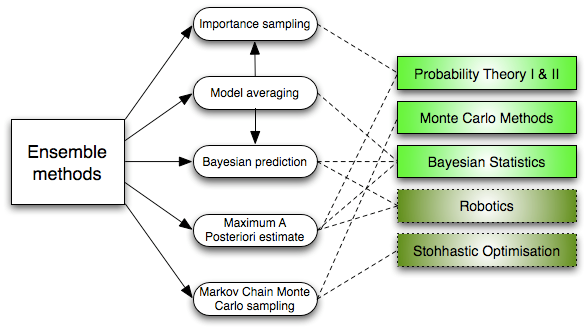Ensemble Learning Algorithms

So how do ensemble methods work and why are they superior to individual models?

• They average out biases: If you average a bunch of democratic-leaning polls and republican-leaning polls together, you will get an average something that isn’t leaning either way.
• They reduce the variance: The aggregate opinion of a bunch of models is less noisy than the single opinion of one of the models. In finance, this is called diversification — a mixed portfolio of many stocks will be much less variable than just one of the stocks alone. This is why your models will be better with more data points rather than fewer.
• They are unlikely to over-fit: If you have individual models that didn’t over-fit, and you are combining the predictions from each model in a simple way (average, weighted average, logistic regression), then there’s no room for over-fitting.

### Unsupervised Learning

7. Clustering Algorithms: Clustering is the task of grouping a set of objects such that objects in the same group (cluster) are more similar to each other than to those in other groups.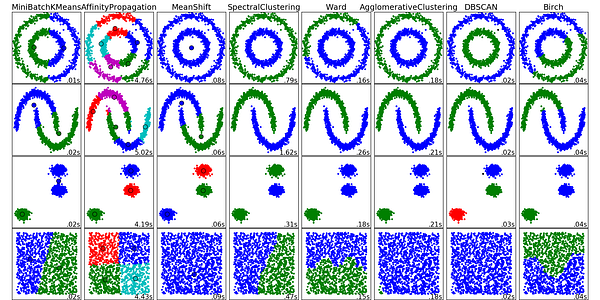Clustering Algorithms

Every clustering algorithm is different, and here are a couple of them:

• Centroid-based algorithms
• Connectivity-based algorithms
• Density-based algorithms
• Probabilistic
• Dimensionality Reduction
• Neural networks / Deep Learning

8. Principal Component Analysis: PCA is a statistical procedure that uses an orthogonal transformation to convert a set of observations of possibly correlated variables into a set of values of linearly uncorrelated variables called principal components.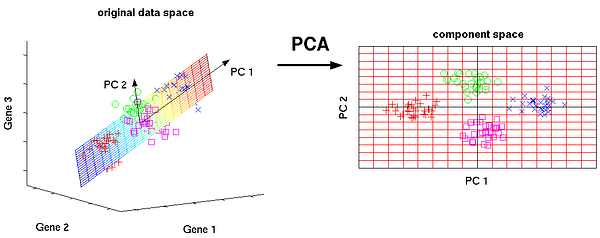Principal Component Analysis

Some of the applications of PCA include compression, simplifying data for easier learning, visualization. Notice that domain knowledge is very important while choosing whether to go forward with PCA or not. It is not suitable in cases where data is noisy (all the components of PCA have quite a high variance).

9. Singular Value Decomposition: In linear algebra, SVD is a factorization of a real complex matrix. For a given m * n matrix M, there exists a decomposition such that M = UΣV, where U and V are unitary matrices and Σ is a diagonal matrix.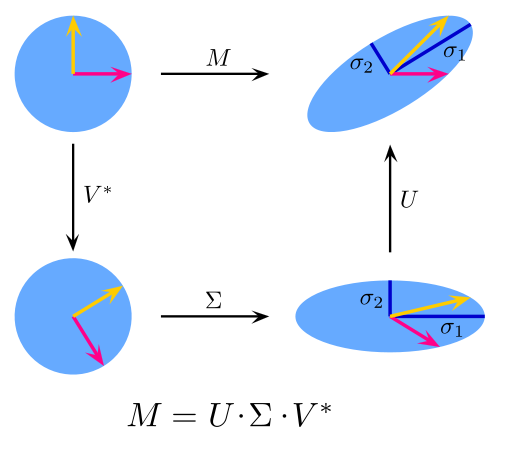Singular Value Decomposition

PCA is actually a simple application of SVD. In computer vision, the 1st face recognition algorithms used PCA and SVD in order to represent faces as a linear combination of “eigenfaces”, do dimensionality reduction, and then match faces to identities via simple methods; although modern methods are much more sophisticated, many still depend on similar techniques.

10. Independent Component Analysis: ICA is a statistical technique for revealing hidden factors that underlie sets of random variables, measurements, or signals. ICA defines a generative model for the observed multivariate data, which is typically given as a large database of samples. In the model, the data variables are assumed to be linear mixtures of some unknown latent variables, and the mixing system is also unknown. The latent variables are assumed non-gaussian and mutually independent, and they are called independent components of the observed data.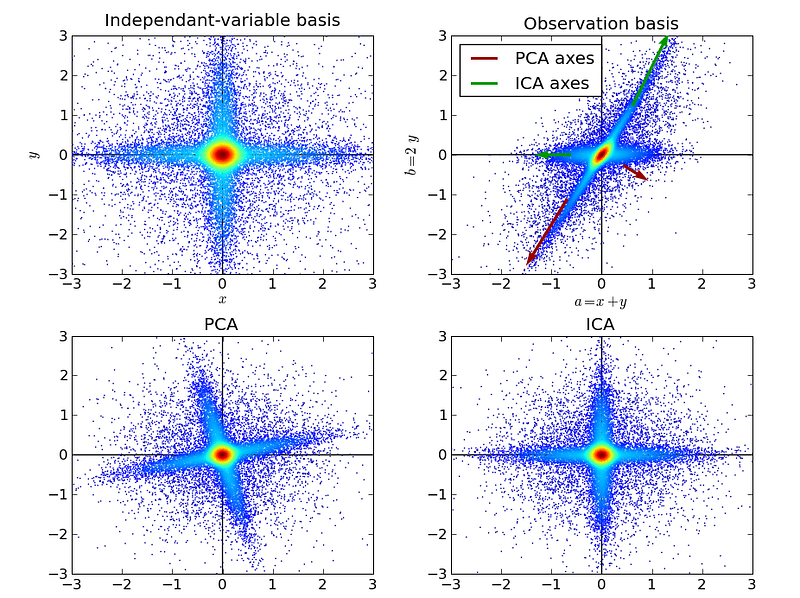Independent Component Analysis

ICA is related to PCA, but it is a much more powerful technique that is capable of finding the underlying factors of sources when these classic methods fail completely. Its applications include digital images, document databases, economic indicators and psychometric measurements.

Now go forth and wield your understanding of algorithms to create machine learning applications that make better experiences for people everywhere.

Bio: James Le is a Product Intern at New Story Charity and a Computer Science and Communication student at Denison University.

Original. Reposted with permission.

Related:Get KDnuggets, a leading newsletter on AI, Data Science, and Machine Learning